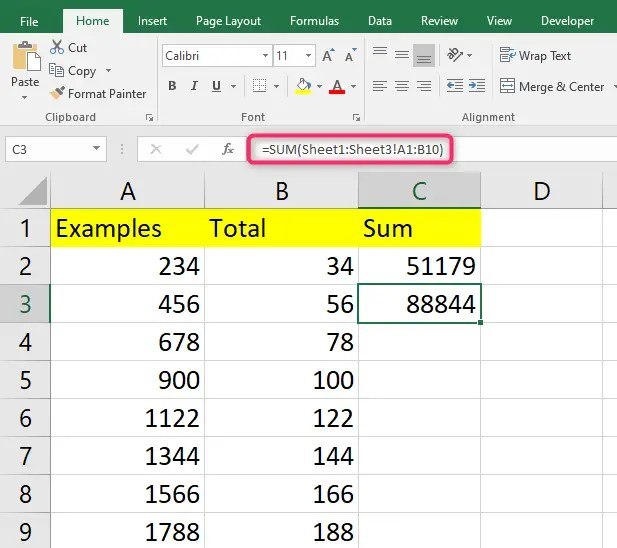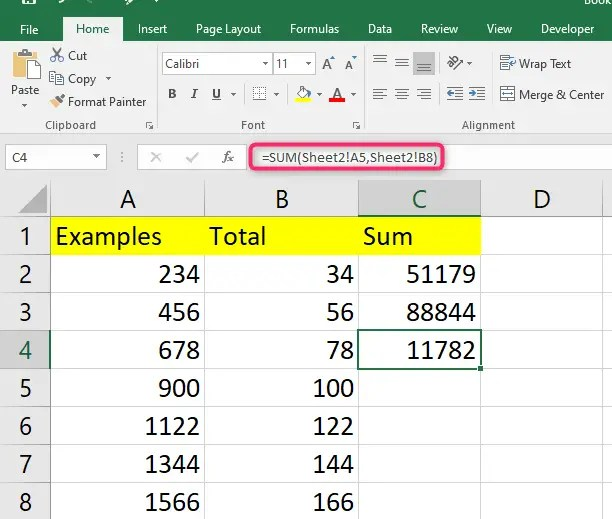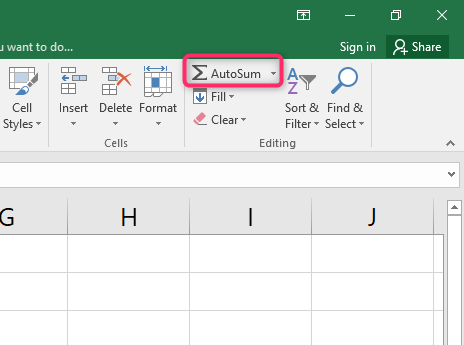# How to sum in Excel between sheets

Summing up data is a normal occurrence when dealing with Excel data. Sometimes you may need to sum up data in cells across different or multiple spreadsheets. It is easy enough to do this using Excel’s Sum formula, where you will reference those cells you want to sum.In this guide, we give you steps to follow when you want to sum in Excel between sheets. Let’s get started.

## Using the Sum function to reference another sheet

You can easily reference another sheet outside of the sheet you are in using Excel’s Sum function.

Select all cells you want to add from another worksheet. To do this, click on them while holding the Ctrl key.

Go to your Sum formula and type in the name of the sheet you want to reference manually. You can find this name in your document by checking the bottom tabs in your Excel workbook.

Change the Excel Sum formula so that multiple sheets can be referenced altogether. To do this, add the name of the specific sheets, follow this with an exclamation point, and lastly, add the name of the cell you want to add. Here is an example of what your formula will look like: =SUM (Sheet1! A20, Sheet2! B24).Now you have referenced another sheet in your Excel Sum formula. You can do this to add multiple sheets to your Sum function.

## How to sum a range in Excel between two sheets

You can also add multiple cells from multiple sheets in your Excel Sum function.

To do this, determine the range of sheets you want to reference by separating them with a colon in the Sum formula. It will be like this =SUM (Sheet1:Sheet10! Cell#). Such a formula will reference all of the sheets in a range and the corresponding cell across every sheet you want to reference.

You can also reference a range of cells to add up across all sheets. To do this, your formula will look like this: =SUM (Sheet1:Sheet3! A1:B10). This formula adds all the cells from A1 through B10 across sheet 1 to sheet 3.## Using the Sum function to find an Excel Sum from another sheet

You can find an Excel Sum from another sheet by picking a cell where you want the total sum formula to go. You can choose a cell in any of the sheets in your Excel workbook.

Format your Sum formula to reference the other sheet with the cell or range of cells you want to add to the formula.

When adding cells located in a different worksheet, start by typing the Sum function =SUM ( followed by the cell located in another worksheet. Afterward, type a comma And select any other cell. Do this to include all cells you want to include in the sum function.## Using the Sum Function to Add cells in the same sheet

In an open Excel worksheet, click on the AutoSum option located in the Editing group of the main menu ribbon.To use the Sum formula, click the first open cell at the bottom of the column you want to add.

Then click the AutoSum button to insert your Sum function. Click the Enter button to tally the sum of cells in the column you chose.